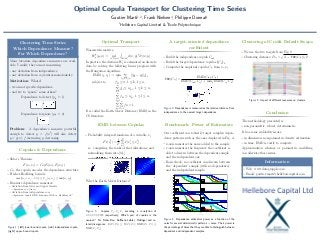Ce diaporama a bien été signalé.

# Optimal Copula Transport for Clustering Time Series

0

PartagerChargement dans…3
×
1 sur 1
1 sur 1

# Optimal Copula Transport for Clustering Time Series

0

Partager

This poster describes the Target Dependence Coefficient.
This coefficient is designed to measure the dependence between random variables. Unlike other dependence measures which simply estimate the distance between the joint distribution and the distribution of independence (i.e. the product of marginals), this coefficient can target specific dependence relationships or forget others. To do so, dependence is measured as the relative distance from the forget-dependence (it can be independence) to the target-dependence (it can be comonotonicity, as usually considered). Earth Mover Distance (discrete optimal transport) is used to evaluate the distance between the different copulas encoding the data-dependence, target-dependence, forget-dependence. Finally, we use this methodology for clustering financial time series (5-year credit default swaps) and observe that it yields more stable clusters than using Pearson, Spearman or Kendall correlation.

This poster describes the Target Dependence Coefficient.
This coefficient is designed to measure the dependence between random variables. Unlike other dependence measures which simply estimate the distance between the joint distribution and the distribution of independence (i.e. the product of marginals), this coefficient can target specific dependence relationships or forget others. To do so, dependence is measured as the relative distance from the forget-dependence (it can be independence) to the target-dependence (it can be comonotonicity, as usually considered). Earth Mover Distance (discrete optimal transport) is used to evaluate the distance between the different copulas encoding the data-dependence, target-dependence, forget-dependence. Finally, we use this methodology for clustering financial time series (5-year credit default swaps) and observe that it yields more stable clusters than using Pearson, Spearman or Kendall correlation.

## Plus De Contenu Connexe

### Livres associés

Gratuit avec un essai de 14 jours de Scribd

Tout voir

### Livres audio associés

Gratuit avec un essai de 14 jours de Scribd

Tout voir

### Optimal Copula Transport for Clustering Time Series

1. 1. Optimal Copula Transport for Clustering Time Series Gautier Marti1,2 , Frank Nielsen2 , Philippe Donnat1 1 Hellebore Capital Limited & 2 Ecole Polytechnique Clustering Time Series Which Dependence Measure? For Which Dependence? Many bivariate dependence measures are avail- able. Usually, they aim at measuring: • any deviation from independence, • any deviation from co/counter-monotonicity. Motivation: What if • we aim at speciﬁc dependence, • and try to “ignore” some others? Dependence to detect (ρij := 1) Dependence to ignore (ρij := 0) Problem: A dependence measure powerful enough to detect y = f(x2 ) will also detect y = g(x), f increasing, g decreasing. Copulas & Dependence • Sklar’s Theorem: F(xi, xj) = Cij(Fi(xi), Fj(xj)) • Cij, the copula, encodes the dependence structure • Fréchet-Hoeﬀding bounds: max{ui + uj − 1, 0} ≤ Cij(ui, uj) ≤ min{ui, uj} • Bivariate dependence measures: • deviation from lower and upper bounds • Spearman’s ρS, Gini’s γ • deviation from independence uiuj • Spearman, Copula MMD, Schweizer-Wolﬀ’s σ, Hoeﬀding’s Φ2 Figure 1: (left) lower-bound copula, (mid) independence copula, (right) upper-bound copula Optimal Transport Wasserstein metrics: Wp p (µ, ν) := inf γ∈Γ(µ,ν) M×M d(x, y)p dγ(x, y) In practice, the distance W1 is estimated on discrete data by solving the following linear program with the Hungarian algorithm: EMD(s1, s2) := min f 1≤k,l≤n pk − ql fkl subject to fkl ≥ 0, 1 ≤ k, l ≤ n, n l=1 fkl ≤ wpk , 1 ≤ k ≤ n, n k=1 fkl ≤ wql , 1 ≤ l ≤ n, n k=1 n l=1 fkl = 1. It is called the Earth Mover Distance (EMD) in the CS literature. A target-oriented dependence coeﬃcient • Build the independence copula Cind • Build the target-dependence copulas {Ck}k • Compute the empirical copula Cij from xi, xj TDC(Cij) = EMD(Cind, Cij) EMD(Cind, Cij) + mink EMD(Cij, Ck) Figure 2: Dependence is measured as the relative distance from independence to the nearest target-dependence EMD between Copulas • Probability integral transform of a variable xi: FT (xk i ) = 1 T T t=1 I(xt i ≤ xk i ), i.e. computing the ranks of the realizations, and normalizing them into [0,1] Why the Earth Mover Distance? Figure 3: Copulas C1, C2, C3 encoding a correlation of 0.5, 0.99, 0.9999 respectively; Which pair of copulas is the nearest? For Fisher-Rao, Kullback-Leibler, Hellinger and re- lated divergences: D(C1, C2) ≤ D(C2, C3); EMD(C2, C3) ≤ EMD(C1, C2) Benchmark: Power of Estimators Our coeﬃcient can robustly target complex depen- dence patterns such as the ones displayed in Fig. 4. • x-axis measures the noise added to the sample • y-axis measures the frequency the coeﬃcient is able to discern between the dependent sample and the independent one • Basic check: no coeﬃcient can discern between the “dependent” sample (with no dependence) and the independent sample. 0.00.40.8 xvals power.cor[typ,] xvals power.cor[typ,] 0.00.40.8 xvals power.cor[typ,] xvals power.cor[typ,] cor dCor MIC ACE RDC TDC 0.00.40.8 xvals power.cor[typ,] xvals power.cor[typ,] 0 20 40 60 80 100 0.00.40.8 xvals power.cor[typ,] 0 20 40 60 80 100 xvals power.cor[typ,] Noise Level Power Figure 4: Dependence estimators power as a function of the noise for several deterministic patterns + noise. Their power is the percentage of times that they are able to distinguish between dependent and independent samples. Clustering of Credit Default Swaps • We use the two targets from Fig. 2 • Clustering distance: Dij = (1 − TDC(Cij))/2 Figure 5: Impact of diﬀerent measures on clusters Conclusion The methodology presented is • non-parametric, robust, deterministic. It has some scalability issues: • in dimension, non-parametric density estimation; • in time, EMD is costly to compute. Approximation schemes or parametric modelling can alleviate these issues. Information • Web: www.datagrapple.com • Email: gautier.marti@helleborecapital.com